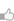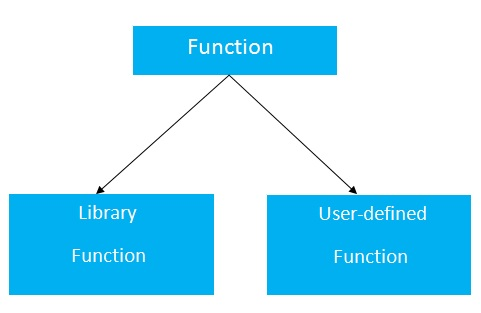求知 文章 文库 Lib 视频 iPerson 课程 认证 咨询 工具 讲座 Modeler Code

 C语言教程 C语言历史 C语言特点 C语言VS开发环境安装 C语言第一个程序 C语言程序执行流程 C语言printf()和scanf()函数 C语言变量 C语言数据类型 C语言关键字 C语言运算符 C语言注释 C语言转义序列 C语言常量 C语言控制语句 C语言if-else语句 C语言switch语句 C语言循环 C语言do-while循环 C语言while循环 C语言for循环 C语言break语句 C语言continue语句 C语言goto语句 C语言类型转换 C语言函数 C语言函数 C语言通过值和引用函数 C语言递归 C语言存储分类 C语言数组 C语言数组 C语言二维数组 C语言将数组传递给函数 C语言指针 C语言指针 C语言指针的指针 C语言指针算术运算 C语言字符串 C语言字符串 C语言gets()和puts()函数 C语言字符串函数 C语言strlen()函数 C语言strcpy()函数 C语言strcat()函数 C语言strcmp()函数 C语言strrev()函数 C语言strlwr()函数 C语言strupr()函数 结构联合体 C语言结构体 C语言结构体数组 C语言结构体嵌套 C语言联合体 文件处理 C语言文件处理 C语言fprintf()和fscanf()函数 C语言fputc()和fgetc()函数 C语言fputs()和fgets()函数 C语言fseek()函数 C语言rewind()函数 C语言ftell()函数 预处理器 C语言预处理器指令 C语言宏 C语言#include指令 C语言#define指令 C语言#undef指令 C语言#ifdef指令 C语言#ifndef指令 C语言#if指令 C语言#error指令 C语言#pragma指令 其它杂项 C语言数学函数 C语言命令行参数
C语言函数
 218 次浏览1次捐助

C语言中的函数在其他编程语言中也称为过程或子程序。要执行任务，我们可以创建函数。一个函数可以被多次调用。 它提供了模块化和代码可重用性。

C语言中函数的优点

(1)代码可重用性

(2)代码优化

C语言编程中有两种类型的函数：C语言中创建函数的语法如下：

 return_type function_name(data_type parameter...){ //code to be executed }

C语言函数可以返回或不返回该函数的值。如果您不需要从函数返回任何值，请对返回类型使用void。

 void hello(){ printf("hello c");// 没有返回值，只是打印一个字符串 }

 int get(){ return 10; }

 float get(){ return 10.2; }

C语言函数可能有0个或更多个参数。可以在C程序中具有任何类型的参数，如：int，float，char等。参数也称为形式参数。

 void hello(){ printf("hello c"); }

 int cube(int n){ return n*n*n; }

 int add(int a, int b){ return a+b; }

 variable=function_name(arguments...);

(1)variable：变量不是强制性的。如果函数返回类型为void，则不能提供变量来接收函数返回的值，因为void函数不返回任何值。(2)function_name：function_name是要调用的函数的名称。(3)arguments：调用C语言函数时需要提供参数。它也被称为实际参数(简称：实参)。

 hello(); //calls function that doesn't return a value int value=get(); //calls function that returns value int value2=add(10,20); //calls parameterized function by passing 2 values

1. 没有return语句的函数示例

 #include #include //定义一个无参数函数，简单地打印一个字符串 void hello() { printf("hello c programming"); } void main() { hello(); // 调用函数 hello(); // 再一次调用函数 hello(); // 再一次调用函数 }

 hello c programming hello c programming hello c programming

2. 带有return语句的C函数示例

 #include // 定义一函数，返回给定参数值的立方值 int cube(int n) { return n*n*n; } // 定义一函数，返回给定两个参数值的最大值 int max(int a, int b) { if (a > b) { return a; }else { return b; } } void main() { int result1 = 0, result2 = 0; int a = 10, b = 20, c = 0; result1 = cube(2); // 调用函数 result2 = cube(5); c = max(a, b); // printf("the cube of 2 is : %d \n", result1); printf("the cube of 5 is : %d \n", result2); printf("the max value of %d and %d is :%d \n", a, b, c); }

 the cube of 2 is : 8 the cube of 5 is : 125 the max value of 10 and 20 is :20

 您可以捐助，支持我们的公益事业。 1元 10元 50元 认证码：必填
 218 次浏览1次捐助
 < 首页